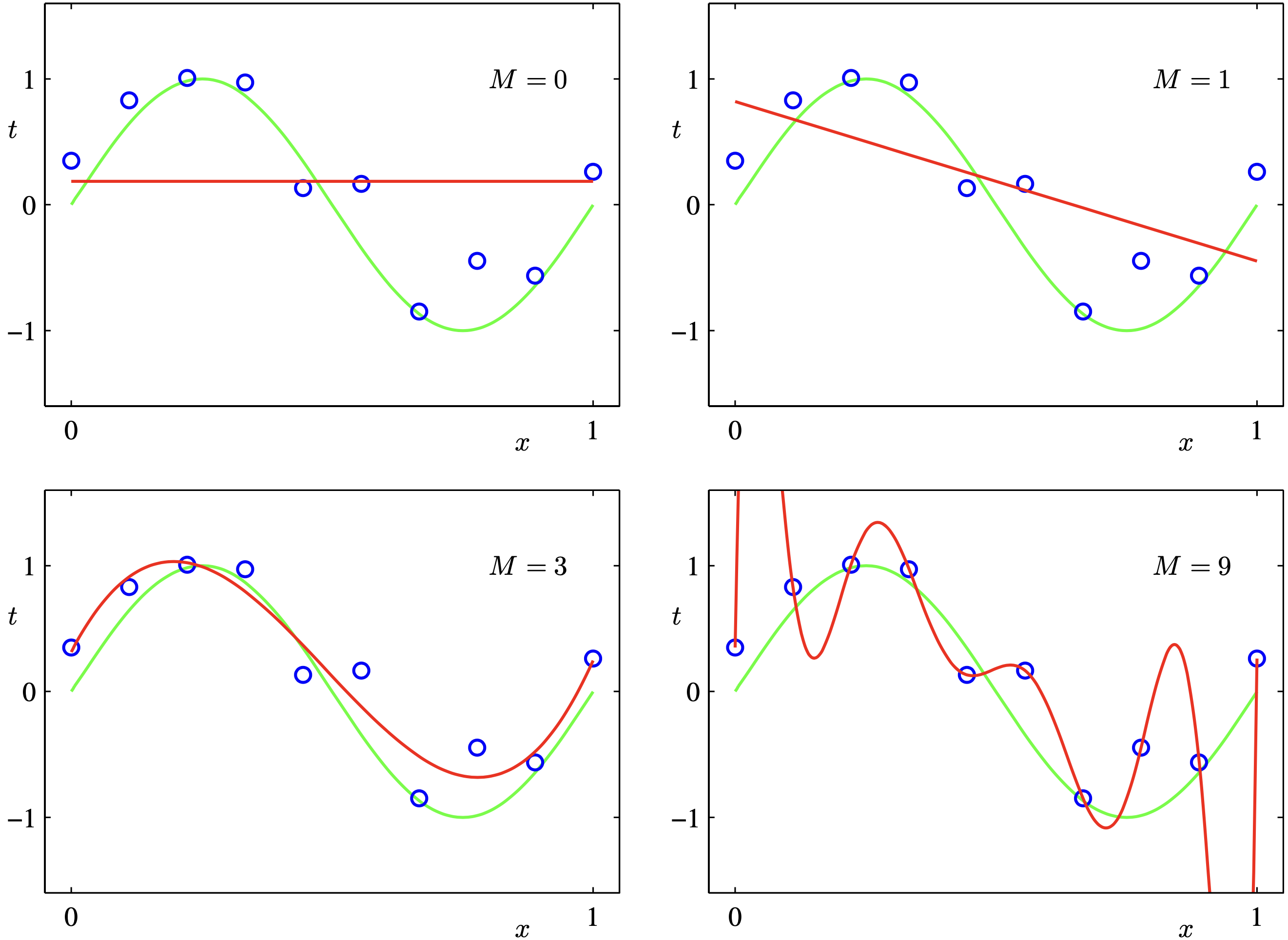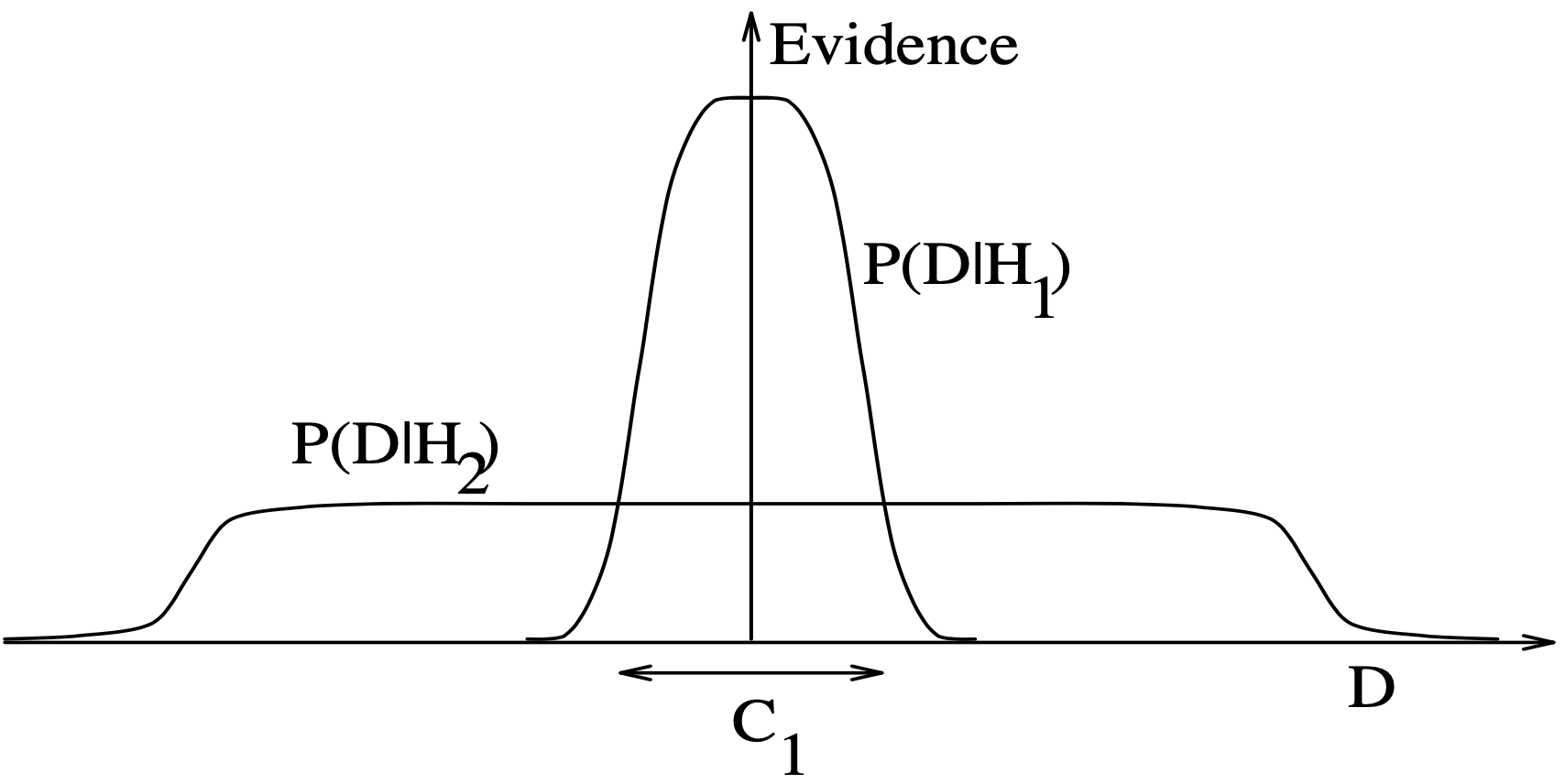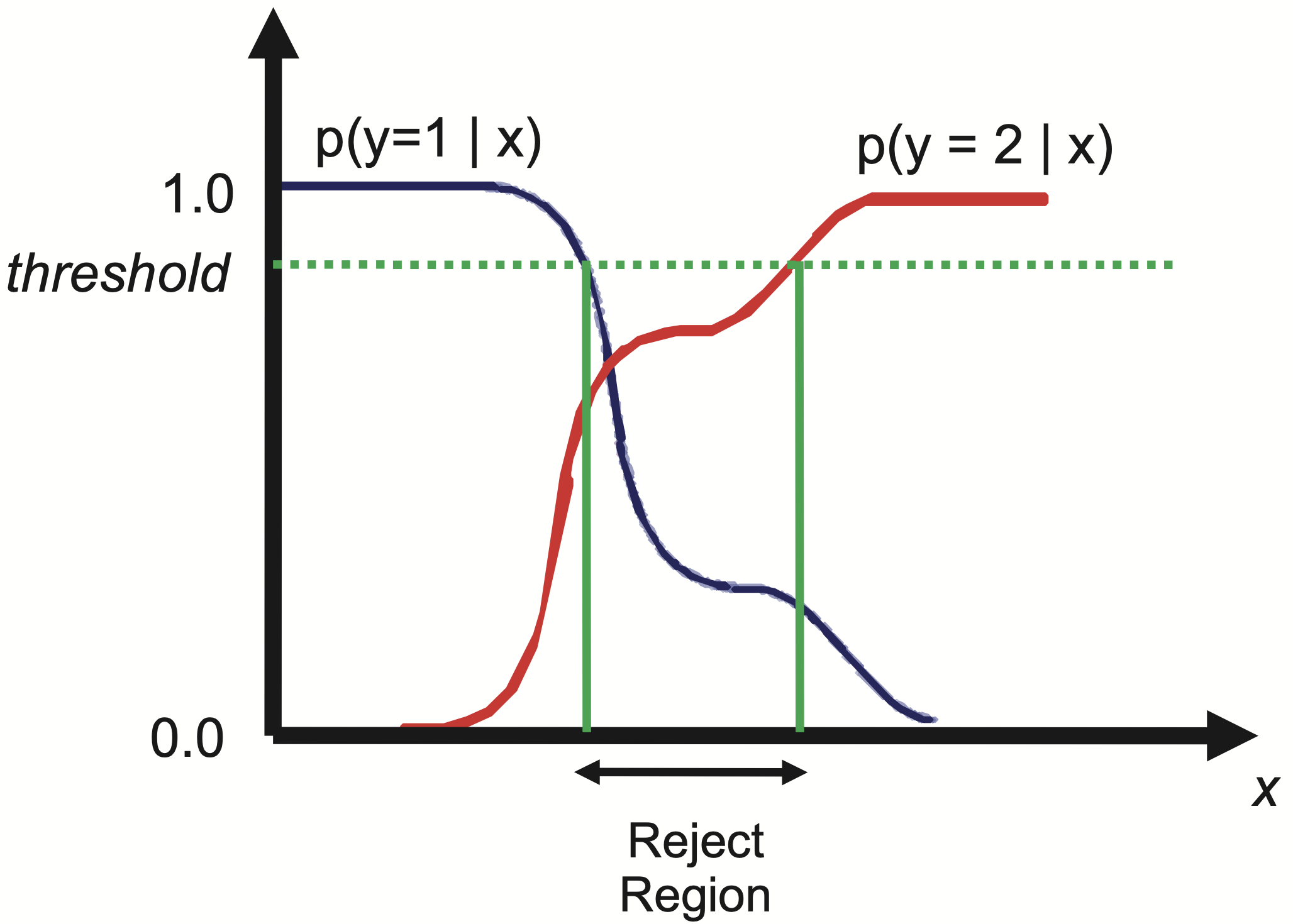The following materials is based on the Chapter 05 of Machine Learning: A Probabilistic Approach and some examples from (Mackay, 2003)1 and (Bishop, 2006)2.

Discussion highlights

1. Posterior distribution (page 149): Using the posterior distribution $$p(\theta\mid\mathcal{D})$$ to summarize everything we know about a set of unknown variables is at the core of Bayesian statistics.
• Prior of $$\theta$$: $$p(\theta)$$
• Likelihood of $$\theta$$: $$p(\mathcal{D}\mid\theta)$$
• Posterior: $p(\theta\mid\mathcal{D}) = \frac{p(\mathcal{D}\mid\theta)p(\theta)}{p(\mathcal{D})} = \frac{p(\mathcal{D}\mid\theta)p(\theta)}{\int_{\theta} p(\mathcal{D}\mid\theta)p(\theta) d\theta}$
• The challenge of estimating $$p(\theta\mid\mathcal{D})$$ is the computation of $$\int_{\theta} p(\mathcal{D}\mid\theta)~p(\theta) d\theta$$
2. Maximum a posteriori estimation (page 149 - 150): MAP estimate identifies the mode of the posterior $\hat{\theta} = \text{argmax}_{\theta} p(\theta\mid\mathcal{D}) = \text{argmax}_{\theta} p(\mathcal{D}\mid\theta) p(\theta)$
• The existence of efficient algorithms: (1) essentially, this is an optimization problem; (2) no need to compute $$p(\mathcal{D})$$
• The connection with regularization. For example, in linear regression, $$\ell_2$$ regularization is equivalent to the Bayesian linear regression with a standard Gaussian prior on $$\theta$$, $$\mathcal{N}(\theta\mid 0, \sigma^2I)$$.
• Issues of MAP: (1) no measure of uncertainty; (2) without no uncertainty of model parameters, predictive distributions could be over-confident; (3) the mode can be untypical point — the mode is a point of measure zero, whereas the mean and median take the volume of the space into account.
3. Model selection (page 155):
• Model selection problem: when faced with a set of models (i.e., families of parameteric distributions) of different complexity, how should we choose the best one?
• Example: fitting data from the function $$\sin(2\pi x)$$ with polynomial functions with degree $$M$$functions with high complexity (e.g., $$M=9$$) have potential to fit more complex data and could exhibit over-fitting on simple datasets (reprinted from Chapter 01 of (Bishop, 2006)2)
• Cross validation: estimate the models on $$K$$-fold of the validation data
• Bayesian model selection: $p(m\mid \mathcal{D})\propto p(\mathcal{D}\mid m) p(m),$ then $$p(m)\propto 1$$, model selection can be done with only $p(\mathcal{D}\mid m)\propto \int_{\theta} p(\mathcal{D}\mid\theta)p(\theta\mid m)d\theta,$ which is called the evidence for model $$m$$.
• Justification of Bayesian model selection: Consider two models $$m_1$$ and $$m_2$$, where model $$m_2$$ has higher complexity than $$m_1$$. For the same data $$\mathcal{D}$$, if both $$m_1$$ and $$m_2$$ can fit $$\mathcal{D}$$, Bayesian model selection will suggest to pick $$m_1$$ as illustrated in the following plot (reprinted from the Chapter 28 of (Mackay, 2003)1 with $$H_1$$ and $$H_2$$ represent the models)4. Non-informative priors (page 165):
• If we don’t have strong beliefs about what $$\theta$$ should be, it is common to use an uninformative or non-informative prior, and to “let the dat speak for itself”.
• If $$\theta$$ is discrete and has $$K$$ states, then $$p(\theta) = \frac{1}{K}$$ is a non-informative prior.
5. Bayesian decision theory (page 176)
• Action: $$a\in\mathcal{A}$$, where $$\mathcal{A}$$ is the action space
• (Ground-truth) label $$y\in\mathcal{Y}$$, where $$\mathcal{Y}$$ is the label space
• Loss $$L(y,a)$$: the loss incurred if we pick action $$a$$ when the ground-truth label is $$y$$
• Example: in classification problems, we often assume $$\mathcal{A}=\mathcal{Y}$$, and $$a$$ as the predicted label $$\hat{y}$$. The popular 0-1 loss function is defined as $L(\hat{y},y) = 1$ if $$\hat{y}\not= y$$; $$L=0$$ when $$\hat{y}=y$$.
• In general, Bayesian decision theory pick an action based on the the minimal expected loss $\delta(x) = \text{argmin}_{a\in\mathcal{A}} E[L(y,a)]$ where $$x$$ is the input.
• With a predictive distribution of $$y$$ given $$x$$, the expected loss in the previous item is defined as $E[L(y,a)] = \sum_{a\in\mathcal{A}} L(y,a)p(y\mid x)$ when we use the 0-1 loss, then the decision rule reduces to the simple classification rule.
• Reject option: In classification problems where $$p(y\mid x)$$ is very uncertain, we may prefer to choose a reject action, in which we refuse to classify the example as any of the specified classes, and instead say “don’t know”.